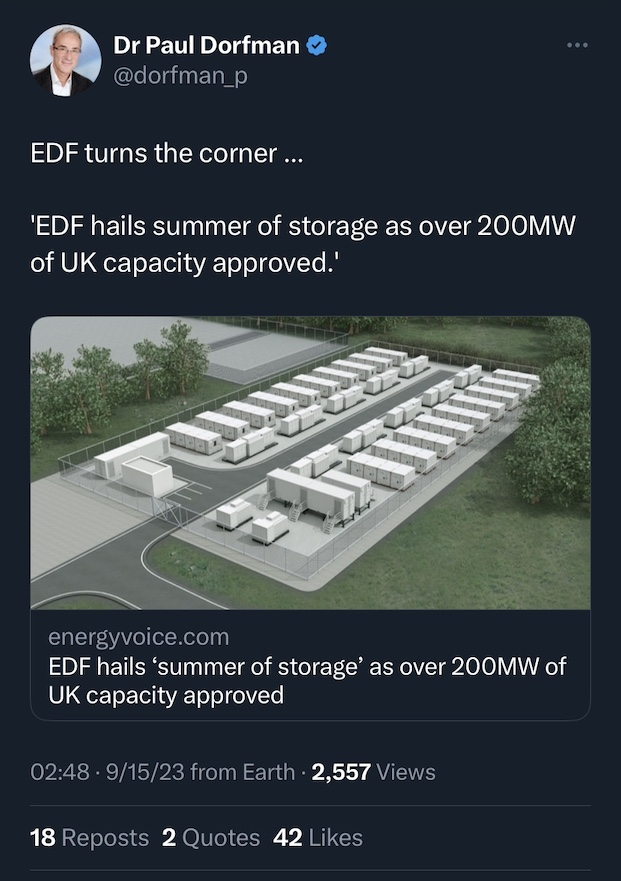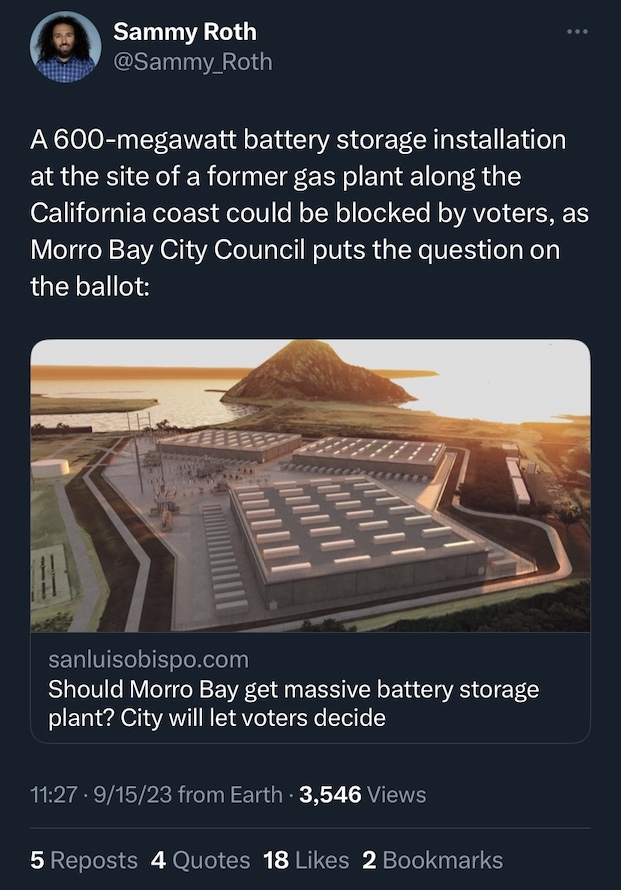# The difference between power and energy

posted: September 16, 2023

tl;dr: If you are measuring the energy capacity of anything in terms of watts, you are confusing power and energy...

Many people use the terms “power” and “energy” interchangeably. For example, I can say “I am a proponent of solar power” or I can say “I am a proponent of solar energy” and my meaning is clear: I like systems which convert energy from the sun into electricity or another useful form of energy. Yet there are many situations where it is important to distinguish between power and energy.

Battery capacity is one such situation. The most important thing that a battery does is store energy for later use. The capacity of a battery (or battery system) is a measure of the total amount of energy the battery can store and then deliver later, when it is needed. But if you are quoting the capacity of a battery in MW (MegaWatts, or millions of watts), you are making a mistake: you are confusing power with energy.

Power is a rate: it is the rate at which the energy of a system changes. A “watt” is the most commonly used unit for measuring power. Power is the rate at which energy flows into or out of a system. As such, power is useful for measuring the rate at which energy is generated or consumed. Mathematically, it is the first derivative of the graph of the energy of a system over time. But measuring power is not measuring energy: they are two different things.Watts measure power, not the energy storage capacity of a battery system

Let’s say you buy a new Tesla EV, and someone asks you, “What is the range of your car?” If you answer “It can go 100 miles per hour!”, you have provided a rate instead of an amount. The questioner wants to know the amount of miles the car can go before needing a charge, not the miles per hour the car can reach. Can it go 100 miles per hour for one hour before needing a charge? Two hours? Five hours? Twenty-four hours?

A battery stores energy like a bucket stores water. The energy storage capacity of the battery is analogous to how much water the bucket can hold. The power rating of the battery is analogous to how quickly water flows out of the bucket when a spigot at the bottom is fully opened. Just as the most important characteristic of a bucket is how much water it holds, the most important characteristic of a battery is how much energy it stores.

Do you know what a joule is? Do you know how to pronounce the word “joule” (answer: it rhymes with “cool”)? If not, you probably confuse power with energy. Joule is the unit that scientists and engineers use to measure energy. If the amount of energy in a system is decreased by one joule in one second, then the power that flowed out of the system was one watt. A watt is a joule per second. A watt is a rate which measures power, whereas a joule is a measure of energy.

In everyday language, one does not often hear the word “joule”. The word “watt” is in common usage because many electrical consumer products (e.g. light bulbs, microwave ovens) come with a watt rating that is a measure of how much power they consume. Multiply one watt by one second and you get one joule, or “watt-second”. But that’s still not a convenient term, because we usually operate electrical devices for longer than one second. So the energy unit that is in common usage is a “watt-hour” (one watt for one hour), or a kilowatt-hour (KWh, 1000 watts for one hour), or when measuring high capacity, electrical grid-scale systems, megawatt-hour or gigawatt-hour. Those are the units that can be used to measure the energy storage capacity of a battery system, if you can't wrap your head around "joules".Watts measure power, not the energy storage capacity of a battery system

If you see battery capacity stated in MW, it is hard to know what that means. Did someone just make a typo by omitting the “h”, and the correct meaning is “MWh” instead of “MW”? Or is the MW figure a measure of the maximum power that can be drawn from the battery system when it is discharged? But then for how long can that power be drained before the battery is depleted? By the way, another characteristic of battery systems that matters is the rate at which they can be charged, i.e. the maximum power that they will accept and store as energy. This, along with the battery system’s energy capacity, determines how long it takes to charge the battery system.

The most important characteristic of a battery system is how much energy it stores, which should be measured in a unit of energy: GWh, MWh, KWh, or joules if you are a nerd scientist or engineer. The maximum discharge power should be measured in watts, as is the maximum charge power. A grid scale battery system with a capacity of 1000MWh can, if it performs well, provide 1000MW (or 1GW) for an hour, or 200MW for 5 hours, or 100MW for 10 hours, or 41.67MW over the course of 24 hours, before being depleted.

Power is the rate at which the energy of a system changes. Hopefully this helps those who are confused understand the difference between power and energy.

Related post: Let’s play Amateur EE!